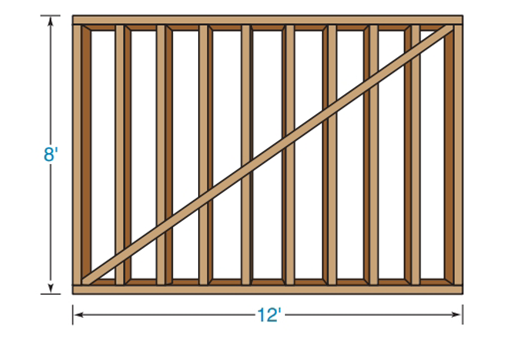Chapter 4.3, Problem 40EElementary Geometry For College St...

7th Edition
Alexander + 2 others
ISBN: 9781337614085

Solutions

Chapter
SectionElementary Geometry For College St...

7th Edition
Alexander + 2 others
ISBN: 9781337614085
Textbook Problem

In Exercises 40 to 41, you will need to use the square root ( ) function of your calculator.A wall that is 12 ft long by 8 ft high has a triangular brace along the diagonal. Use a calculator to approximate the length of the brace to the nearest tenth of a foot.To determine

To find:

The length of the brace.

Explanation

Calculation:

Consider the rectangle ABCD with a length 12, height 8 and B is a right angle and hence ABC is a right triangle with a hypotenuse AC¯.

By using Pythagorean Theorem

(Brace)2=(length

Still sussing out bartleby?

Check out a sample textbook solution.

See a sample solution

The Solution to Your Study Problems

Bartleby provides explanations to thousands of textbook problems written by our experts, many with advanced degrees!

Get Started

Find the derivative. Simplify where possible. 38. f(t)=1+sinht1sinht

Single Variable Calculus: Early Transcendentals, Volume I

Find the modes of the sets of scores in Problems 9-12. 12. 5, 7, 7, 7, 9, 12, 4, 6, 6, 8

Mathematical Applications for the Management, Life, and Social Sciences

Simplify: 1240

Elementary Technical Mathematics

Find the function f such that f(x) = xf(x) x and f(0) = 2.

Single Variable Calculus: Early Transcendentals

45 15 63 127

Study Guide for Stewart's Multivariable Calculus, 8th